# bar3

•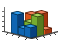## Syntax

``bar3(z)``
``bar3(y,z)``
``bar3(___,width)``
``bar3(___,style)``
``bar3(___,color)``
``bar3(ax,___)``
``b = bar3(___)``

## Description

example

````bar3(z)` creates a 3-D bar graph for the elements of `z`. Each bar corresponds to an element in `z`. To plot a single series of bars, specify `z` as a vector. For a vector of length m, the function plots the bars on a y-axis ranging from `1` to m.To plot multiple series of bars, specify `z` as a matrix with one column for each series. For an m-by-n matrix, the function plots the bars on an x-axis ranging from `1` to n and a y-axis ranging from `1` to m. ```

example

````bar3(y,z)` creates a bar graph of the elements in `z` at the y-values specified in `y`. If `z` is a matrix, elements from the same row in `z` appear at the same location along the y-axis.```

example

````bar3(___,width)` sets the width of the bars along the x- and y-axes and controls the separation of bars within a group. By default, `width` is `0.8` and the bars have a slight separation. If `width` is `1`, the bars within a group touch one another.```

example

````bar3(___,style)` specifies the style of the bars, where `style` can be `'detached'`, `'grouped'`, or `'stacked'`. The default mode of display is `'detached'`.```

example

````bar3(___,color)` displays all bars using the color specified by `color`. For example, use `'r'` to specify all red bars.```

example

````bar3(ax,___)` plots into the axes specified by `ax` instead of into the current axes (`gca`). The option `ax` can precede any of the input argument combinations in the previous syntaxes.```

example

````b = bar3(___)` returns one or more `Surface` objects. If `z` is a vector, then `bar3` creates one `Surface` object. If `z` is a matrix, then `bar3` returns a `Surface` object for each series. Use `b` to set properties of the bars after displaying the bar graph. For a list of properties, see Surface Properties.```

## Examples

collapse all

Specify `z` as a vector of five values. Plot these values as a series of 3-D bars, with the height of each bar corresponding to a value in `z` and its y-axis location corresponding to the index of that value.

```z = [50 40 30 20 10]; bar3(z);```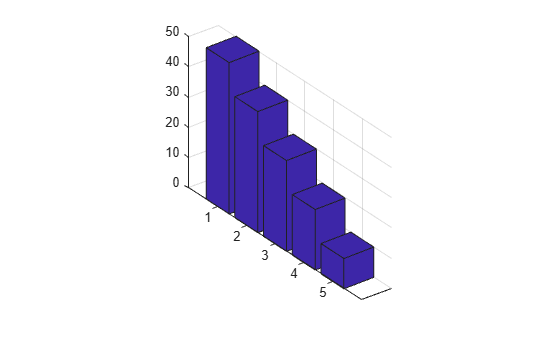Specify `z` as a matrix. Create a 3-D bar graph of `z` with each series corresponding to a column in `z`.

```z = [1 4 7; 2 5 8; 3 6 9; 4 7 10]; bar3(z)```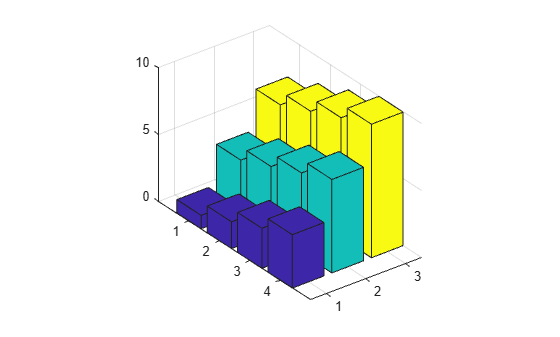Specify `y` as a vector of y-axis locations for the bars in `z`. Plot the bars at the specified y-axis locations.

```y = [1950 1960 1970 1980 1990]; z = [16 8 4 2 1]; bar3(y,z)```Specify `z` as a matrix with three series. Plot `z` with the data for each row grouped together by using the `'grouped'` style. To eliminate space between bars of the same group, set `width` to `1`.

```z = [70 50 33 10; 75 55 35 15; 80 60 40 20]; bar3(z,1,'grouped')```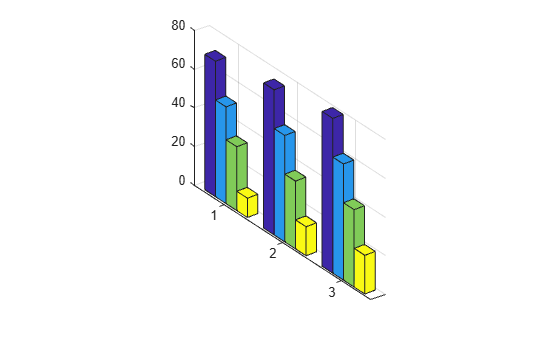Create a set of y-coordinates ranging from `0` to $\pi$. Plot sine functions of the y-values as a 3-D bar graph. Use the color specification `'r'` to make the bars red.

```y = 0:pi/16:pi; z = [sin(y')/4 sin(y')/2 sin(y')]; bar3(y,z,1,'r')```Plot data matrix z with the bar style `'stacked'` and bar width `0.5`. Store the returned `Surface` objects as `b`. In this case, `b` has 4 elements, with one for each column in `z`.

Note that `bar3` colors each series based on the default colormap.

```z = [19 30 21 30; 40 16 32 12]; b = bar3(z,0.5,'stacked');```Customize the color of each series by setting the `FaceColor` of the corresponding object in `b`.

```b(1).FaceColor = 'k'; b(2).FaceColor = 'white'; b(3).FaceColor = [.5 .7 .8]; b(4).FaceColor = [.9 .7 .8];```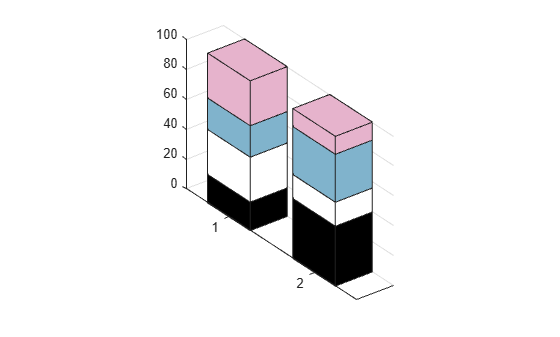Since R2019b

You can display a tiling of plots using the `tiledlayout` and `nexttile` functions. Call the `tiledlayout` function to create a 1-by-2 tiled chart layout. Call the `nexttile` function to create the axes objects `ax1` and `ax2`. Create separate line plots in the axes by specifying the axes object as the first argument to `bar3`.

Display a stacked 3-D bar graph in the left axes. In the right axes, display a grouped bar graph of the same data.

```tiledlayout(1,2) z = [64 56 48; 49 42 35; 36 30 24]; % Left bar graph ax1 = nexttile; bar3(ax1,z,0.5,'stacked') % Right bar graph ax2 = nexttile; bar3(ax2,z,1,'grouped')```## Input Arguments

collapse all

z-coordinates, specified as a vector or matrix. The dimensions of `z` determine how the function displays your data. This table describes the most common situations.

PresentationHow to Specify Y and ZExampleResult
Display one series of bars.
• Specify `y` and `z` as vectors that are the same length. The values in `y` must be unique, but the values in `z` do not need to be unique.

• Alternatively, specify just the `z` values.

Define vectors `y` and `z`, then create a 3-D bar chart.

```y = [1 2 3 4]; z = [10 20 30 40]; bar3(y,z)```

Alternatively, specify just the `z` values.

`bar3(z)`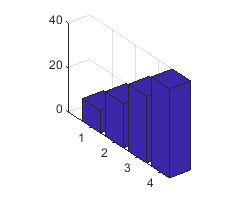Display multiple series of bars stacked along the x-axis.
• Specify `y` as a vector and `z` as a matrix. The number of elements in `y` must match the number of rows in `z`.

• Alternatively, specify just the `z` matrix.

Define vector `y` and matrix `z`, then create a 3-D bar chart with three series of bars.

```y = [1 2 3 4]; z = [1 5 9; 2 6 10; 3 7 11; 4 8 12]; bar3(y,z)```

Alternatively, specify just the `z` values.

`bar3(z)`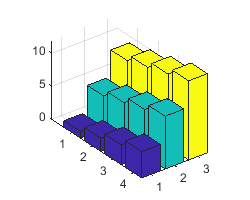Data Types: `single` | `double` | `int8` | `int16` | `int32` | `int64` | `uint8` | `uint16` | `uint32` | `uint64` | `duration`

y-coordinates, specified as a vector. The values of `y` do not need to be in order, but the size of `y` depends on the size of `z` and how you want to display your data. This table describes the most common situations.

PresentationHow to Specify Y and ZExampleResult
Display one series of bars.
• Specify `y` and `z` as vectors that are the same length. The values in `y` must be unique, but the values in `z` do not need to be unique.

• Alternatively, specify just the `z` values.

Define vectors `y` and `z`, then create a 3-D bar chart.

```y = [1 2 3 4]; z = [10 20 30 40]; bar3(y,z)```

Alternatively, specify just the `z` values.

`bar3(z)`Display multiple series of bars stacked along the x-axis.
• Specify `y` as a vector and `z` as a matrix. The number of elements in `y` must match the number of rows in `z`.

• Alternatively, specify just the `z` matrix.

Define vector `y` and matrix `z`, then create a 3-D bar chart with three series of bars.

```y = [1 2 3 4]; z = [1 5 9; 2 6 10; 3 7 11; 4 8 12]; bar3(y,z)```

Alternatively, specify just the `z` values.

`bar3(z)`Data Types: `single` | `double` | `int8` | `int16` | `int32` | `int64` | `uint8` | `uint16` | `uint32` | `uint64` | `categorical` | `datetime` | `duration`

Bar width, specified as a scalar representing a fraction of the total space available for each bar. The default of `0.8` means the bar width is 80% of the space from the previous bar to the next bar, with 10% of that space on each side. If the width is `1`, then the bars within a group touch one another.

Example: `bar3([1 2 3],0.5)` creates bars that use 50% of the available space.

Data Types: `single` | `double` | `int8` | `int16` | `int32` | `int64` | `uint8` | `uint16` | `uint32` | `uint64`

Group style, specified as `'detached'`, `'grouped'`, or `'stacked'`. The group style determines how multiple series of bars display with respect to one another. If `z` is a vector, style does not affect graph appearance.

This table shows the result of each group style option.

Group StyleResultExample

`'detached'`

Display each bar at its corresponding x and y value location.`'grouped'`

Display each group as adjacent bars that are centered around their corresponding y value.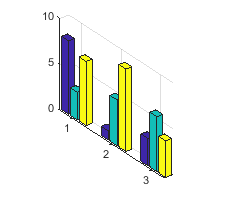`'stacked'`

Display each group as one multicolored bar. The length of a bar is the sum of the elements in the group.Bar color, specified as one of the options in this table.

Color NameShort NameAppearance
`'red'``'r'``'green'``'g'``'blue'``'b'``'cyan'` `'c'``'magenta'``'m'``'yellow'``'y'``'black'``'k'``'white'``'w'`Axes object. If you do not specify an axes, then `bar3` uses the current axes for the bar graph.

## Output Arguments

collapse all

Displayed bars, returned as a vector of `Surface` objects. Use the elements in `b` to access and modify properties of a specific series of bars after it has been created. The number of `Surface` objects returned depends on the size of `z`. If `z` is a vector, then `b` is one `Surface` object. If `z` is a matrix, then `b` is a vector containing a `Surface` object for each column in `z`.

collapse all

### Series of Bars

A series of bars consists of all the bars that correspond to a specific column in `z`. The `bar3` function displays each series at a different location along the x-axis. By default, each series is a different color.

In the following image, the first series is highlighted.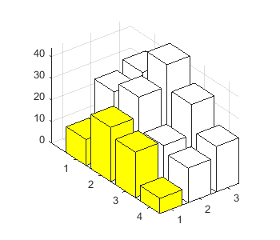### Group of Bars

A group consists of all the bars at a particular y location.

In the following image, the third group is highlighted.## Tips

• The plotted bars can be modified by altering the properties of the returned `Surface` objects. For a list of `Surface` properties, see Surface Properties.

## Version History

Introduced before R2006a

expand all